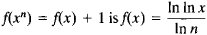# Functional Equation

(redirected from Abel functional equation)
The following article is from The Great Soviet Encyclopedia (1979). It might be outdated or ideologically biased.

## Functional Equation

an equation in which the unknown is a function.

Defined in this way, functional equations form a very large class. Although differential, integral, and finite difference equations are, in principle, functional equations, the term “functional equation” is not usually applied to equations of these types. Functional equations in the narrow sense are equations in which the unknown functions are linked to the given functions of one or more variables by means of the operation of composition of functions. A functional equation can also be viewed as the expression of a property that characterizes a certain class of functions. For example, the functional equation f(x) = f(– x) characterizes the class of even functions, and the functional equation f{x + 1) = f(x) characterizes the class of periodic functions of period 1.

One of the simplest functional equations is the equation f{x + y) = f(x) + f(y). Its continuous solutions are of the form f(x) = Cx. In the class of discontinuous functions, however, this equation has additional solutions. Related to this equation are the functional equations f(x + y) = f(x)f(y), f(xy) = f(x) + f(y), and f(xy) = f(x)f(y); their continuous solutions are eCx, C In x, and xα (x > 0), respectively. Thus, these functional equations determine the exponential, logarithmic, and power functions.

In the theory of analytic functions, functional equations are often used to introduce new classes of functions. For example, doubly periodic functions are characterized by the functional equations f(z + a) = f(z) and f(z + b) = f(z), and automorphic functions are characterized by the functional equations f(sα) = f(z), where {sα} is some group of linear fractional transformations. If a function is defined in a certain domain and satisfies a known functional equation, this equation can be used to extend the domain of definition of the function. Thus, the functional equation f(x + 1) = f(x) for a periodic function enables us to define the function for all x provided that we know its values on the interval [0,1]. This fact is often used for analytic continuation of functions of a complex variable. For example, by using the functional equation Γ(z + 1) = zΓ(z), we can define Γ(z) for all zas soon as we know its values in the strip 0 ≤ Re z ≤ 1 (seeGAMMA FUNCTION).

The symmetry conditions in a physical problem determine the transformation laws of the solutions of the problem for various coordinate transformations. The functional equations that must be satisfied by a solution of the problem are thereby determined. In turn, these functional equations often simplify the task of finding solutions.

Solutions of functional equations can be specific functions or classes of functions, the classes being dependent on arbitrary parameters or functions. For some functional equations, it is possible to obtain the general solution as soon as we know one or more particular solutions. For example, the general solution of the functional equation f(x) = f(ax) is φ[ω(x)], where φ(x) is an arbitrary function and ω(x) is a particular solution of the functional equation. In many cases, a functional equation can be solved by reducing it to a differential equation. This method yields only differentiable solution functions.

Another method of solving functional equations is the method of iteration. It is applicable, for example, to Abel’s equation f[α(x)] = f(x) + 1, where α(x) is a given function, and to the related Schroder equation f[α(x)] = Cf(x). A. N. Korkin showed that if α(x) is analytic then Abel’s equation has an analytic solution. These results were applied in the theory of Lie groups (seeTOPOLOGICAL GROUP), and subsequently led to the development of the iteration theory of analytic functions. In some cases it is possible to obtain solutions of Abel’s equation in closed form. Thus, a particular solution of the functional equation### REFERENCE

Atsel’, Ia. “Nekotorye obshchie metody v teorii funktsional’nykh uravnenii odnoi peremennoi: Novye primeneniia funktsional’nykh uravnenii.” Uspekhi matematicheskikh nauk, 1956, vol. II, issue 3, pp. 3–68.